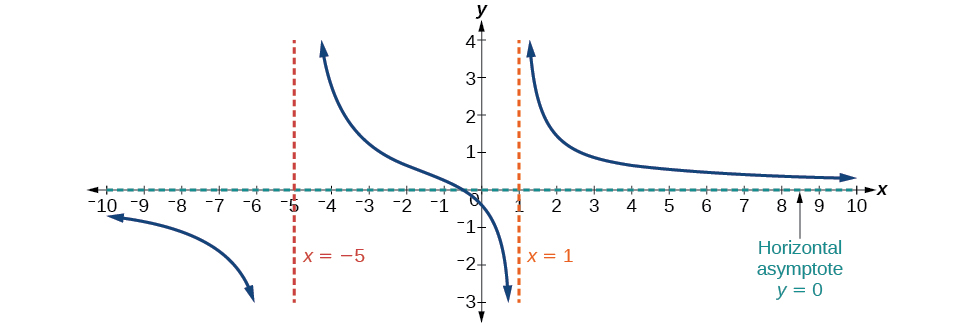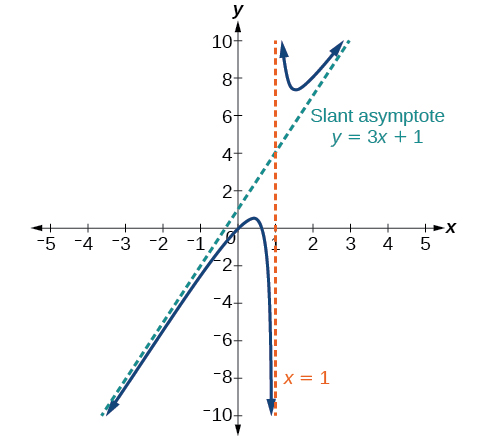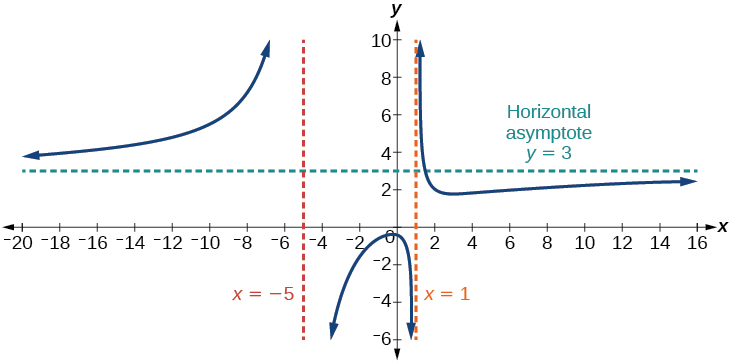# 3.7 Rational functions  (Page 5/16)

 Page 5 / 16

Find the vertical asymptotes and removable discontinuities of the graph of $\text{\hspace{0.17em}}f\left(x\right)=\frac{{x}^{2}-25}{{x}^{3}-6{x}^{2}+5x}.$

Removable discontinuity at $\text{\hspace{0.17em}}x=5.\text{\hspace{0.17em}}$ Vertical asymptotes:

## Identifying horizontal asymptotes of rational functions

While vertical asymptotes describe the behavior of a graph as the output gets very large or very small, horizontal asymptotes help describe the behavior of a graph as the input gets very large or very small. Recall that a polynomial’s end behavior will mirror that of the leading term. Likewise, a rational function’s end behavior will mirror that of the ratio of the leading terms of the numerator and denominator functions.

There are three distinct outcomes when checking for horizontal asymptotes:

Case 1: If the degree of the denominator>degree of the numerator, there is a horizontal asymptote    at $\text{\hspace{0.17em}}y=0.$

In this case, the end behavior is $\text{\hspace{0.17em}}f\left(x\right)\approx \frac{4x}{{x}^{2}}=\frac{4}{x}.\text{\hspace{0.17em}}$ This tells us that, as the inputs increase or decrease without bound, this function will behave similarly to the function $\text{\hspace{0.17em}}g\left(x\right)=\frac{4}{x},\text{\hspace{0.17em}}$ and the outputs will approach zero, resulting in a horizontal asymptote at $\text{\hspace{0.17em}}y=0.\text{\hspace{0.17em}}$ See [link] . Note that this graph crosses the horizontal asymptote.Horizontal Asymptote   y = 0   when   f ( x ) = p ( x ) q ( x ) ,   q ( x ) ≠ 0   where degree of   p < d e g r e e   o f   q .

Case 2: If the degree of the denominator<degree of the numerator by one, we get a slant asymptote.

In this case, the end behavior is $\text{\hspace{0.17em}}f\left(x\right)\approx \frac{3{x}^{2}}{x}=3x.\text{\hspace{0.17em}}$ This tells us that as the inputs increase or decrease without bound, this function will behave similarly to the function $\text{\hspace{0.17em}}g\left(x\right)=3x.\text{\hspace{0.17em}}$ As the inputs grow large, the outputs will grow and not level off, so this graph has no horizontal asymptote. However, the graph of $\text{\hspace{0.17em}}g\left(x\right)=3x\text{\hspace{0.17em}}$ looks like a diagonal line, and since $\text{\hspace{0.17em}}f\text{\hspace{0.17em}}$ will behave similarly to $\text{\hspace{0.17em}}g,\text{\hspace{0.17em}}$ it will approach a line close to $\text{\hspace{0.17em}}y=3x.\text{\hspace{0.17em}}$ This line is a slant asymptote.

To find the equation of the slant asymptote, divide $\text{\hspace{0.17em}}\frac{3{x}^{2}-2x+1}{x-1}.\text{\hspace{0.17em}}$ The quotient is $\text{\hspace{0.17em}}3x+1,\text{\hspace{0.17em}}$ and the remainder is 2. The slant asymptote is the graph of the line $\text{\hspace{0.17em}}g\left(x\right)=3x+1.\text{\hspace{0.17em}}$ See [link] .Slant Asymptote when   f ( x ) = p ( x ) q ( x ) ,   q ( x ) ≠ 0   where degree of   p > degree of  q   by   1 .

Case 3: If the degree of the denominator = degree of the numerator, there is a horizontal asymptote at $\text{\hspace{0.17em}}y=\frac{{a}_{n}}{{b}_{n}},\text{\hspace{0.17em}}$ where $\text{\hspace{0.17em}}{a}_{n}\text{\hspace{0.17em}}$ and $\text{\hspace{0.17em}}{b}_{n}\text{\hspace{0.17em}}$ are the leading coefficients of $\text{\hspace{0.17em}}p\left(x\right)\text{\hspace{0.17em}}$ and $\text{\hspace{0.17em}}q\left(x\right)\text{\hspace{0.17em}}$ for $\text{\hspace{0.17em}}f\left(x\right)=\frac{p\left(x\right)}{q\left(x\right)},q\left(x\right)\ne 0.$

In this case, the end behavior is $\text{\hspace{0.17em}}f\left(x\right)\approx \frac{3{x}^{2}}{{x}^{2}}=3.\text{\hspace{0.17em}}$ This tells us that as the inputs grow large, this function will behave like the function $\text{\hspace{0.17em}}g\left(x\right)=3,\text{\hspace{0.17em}}$ which is a horizontal line. As $\text{\hspace{0.17em}}x\to ±\infty ,f\left(x\right)\to 3,\text{\hspace{0.17em}}$ resulting in a horizontal asymptote at $\text{\hspace{0.17em}}y=3.\text{\hspace{0.17em}}$ See [link] . Note that this graph crosses the horizontal asymptote.Horizontal Asymptote when   f ( x ) = p ( x ) q ( x ) ,   q ( x ) ≠ 0   where degree of  p = degree of  q .

Notice that, while the graph of a rational function will never cross a vertical asymptote    , the graph may or may not cross a horizontal or slant asymptote. Also, although the graph of a rational function may have many vertical asymptotes, the graph will have at most one horizontal (or slant) asymptote.

difference between calculus and pre calculus?
give me an example of a problem so that I can practice answering
x³+y³+z³=42
Robert
dont forget the cube in each variable ;)
Robert
of she solves that, well ... then she has a lot of computational force under her command ....
Walter
what is a function?
I want to learn about the law of exponent
explain this
what is functions?
A mathematical relation such that every input has only one out.
Spiro
yes..it is a relationo of orders pairs of sets one or more input that leads to a exactly one output.
Mubita
Is a rule that assigns to each element X in a set A exactly one element, called F(x), in a set B.
RichieRich
If the plane intersects the cone (either above or below) horizontally, what figure will be created?
can you not take the square root of a negative number
No because a negative times a negative is a positive. No matter what you do you can never multiply the same number by itself and end with a negative
lurverkitten
Actually you can. you get what's called an Imaginary number denoted by i which is represented on the complex plane. The reply above would be correct if we were still confined to the "real" number line.
Liam
Suppose P= {-3,1,3} Q={-3,-2-1} and R= {-2,2,3}.what is the intersection
can I get some pretty basic questions
In what way does set notation relate to function notation
Ama
is precalculus needed to take caculus
It depends on what you already know. Just test yourself with some precalculus questions. If you find them easy, you're good to go.
Spiro
the solution doesn't seem right for this problem
what is the domain of f(x)=x-4/x^2-2x-15 then
x is different from -5&3
Seid
All real x except 5 and - 3
Spiro
***youtu.be/ESxOXfh2Poc
Loree
how to prroved cos⁴x-sin⁴x= cos²x-sin²x are equal
Don't think that you can.
Elliott
By using some imaginary no.
Tanmay
how do you provided cos⁴x-sin⁴x = cos²x-sin²x are equal
What are the question marks for?
ElliottByByBy Edgar DelgadoBy OpenStaxBy Brianna BeckBy OpenStaxBy Jonathan LongBy OpenStaxBy RhodesBy Hoy WenByBy Darlene Paliswat